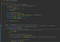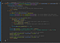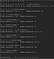# Sorting Algorithms — Quick Sort

Hi I’m Lahiru Abegunawardena. This is my 4th article on “Sorting Algorithms” topic. You can find previous articles on Sorting algorithms and much more interesting topics here. Today we’ll discuss about Quick sort algorithm.

Quick sort is a sorting algorithm which follows divide and conquer method. First of all we need to understand what is this divide and conquer method.

## Divide and conquer method

Think about some problem or a task which has a huge scope, thinking of solving this at onetime is hard and even can’t give the optimum solution. But if you divide this into subtasks with smallest scope, you can solve them one by one and give them the perfect solution.

So like this in divide and conquer algorithm we divide our task into smallest tasks and solve them one by one. So this can help you to get into solution in quicker way.

## How we can apply divide & conquer into sorting ?

Sorting a list of 100 numbers is a huge task, but think if you can divide them into 10 groups. You can solve each group and rearrange those 10 sorted group accordingly.

But if you can do this division in much more planned way you can even get into solution within optimum time. This is the basic idea what we are going to do.

## Quick sort in brief

Think of list of numbers you are going to sort. You are going to need a pivot in order to start divide. Pivot is the value we need to divide number list, normally we write numbers less than pivot in left side and higher numbers into right side. There are few popular methods to choose the pivot out of number list.

1. Last element of the list ( → arraylist.get(0))
2. First element of the list ( → arraylist.get(0))
3. middle item of the list ( → arraylist.get( ((arraylist.size()+1)/2)-1 ))

So every time you divide a number list into to you keep identifying a pivot and continue division until every list contains only one element. After that you can write them according to order from left to right exactly as given below

1. left side list
2. Pivot
3. right side list

Please refer the example I attached below.

## Implementation of Quick Sort algorithmcode Quick Sort — 1code Quick Sort — 2

If you understood these steps already, you should be able to understand by now that we need to use recursive function in order to achieve this task. So first let me explain my recursive function.

public ArrayList<Integer> sortArray(ArrayList<Integer> arr, int firstIndex, int lastIndex) {}

First of all you can see that in parameter list it includes arraylist of integers which contains number list. So when you call function recursive you add your higher or lesser than pivot lists into this. Next parameter in start index most probably this is value 0 and last index which equals to (arraylist.size()-1).

In a recursive function you need to check what’s the check points where you no longer call itself recursively or you do. So in here it means where your arraylist is empty. So that means in that instance this function is called by anotherarray list which only has 1 element(pivot of it). Unless (if arraylist is not empty) you have to identify pivot (in this I use last element) and identify lesser than and higher than elements and call functions recursively. I think you can understand this better with comments I added in code.

Now lets see the result from executionExecution — QuickSort

# Performance

Reference: Quick sort from wikipedia

Let’s look what is time complexity of this.

no of items → n

Worst case → O(n²)

BestCase → O(n logn)

AverageCase → O(n logn)

So as you can see O (nlogn) is the average case which implies this is one of the quickest sorting algorithms. n is for number of items in arraylist.

I hope you will find this article very helpful in learning about Quicksort algorithm and my implementation. I would like to suggest you to try implement sorting by descending order for a better understanding on concept.

Next article will be about another sorting algorithm and its implementation or algorithm explanation. If there is anything to clarify or solve please don’t hesitate to contact me via my LinkedIn and fb page. Try all of this by yourself and if there is anything feel free to comment. Like and please Share with your friends.

## More from Lahiru Sampath Abegunawardena

Software Engineer